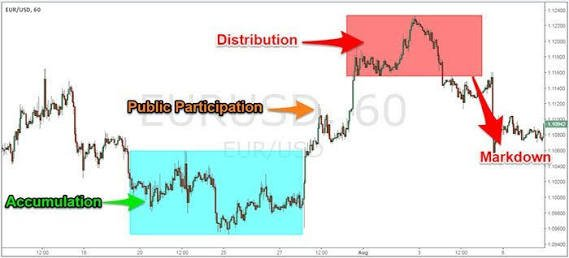## Featured post

### Dow theory : 3 phases of major trends.

There are 3 phases of Dow Theory major trends: 1-  Accumulation phase :- If the previous trend was down then this is the phase where ...## Thursday, December 17, 2015

### CALCULATING EARNING PER SHARE(INCOME STATEMENT), BOOK VALUE(BALANCE SHEET), P/E RATIO AND P/BV RATIO

CALCULATING EARNING PER SHARE(INCOME STATEMENT) AND BOOK VALUE(BALANCE SHEET)

Given:
Market Price: 100,000
Net Income: 20,000
Equity: 7,000
Shares Outstanding(referring total # of shares) = 10,000
Market Price = 10 per share

Answer: Earnings(ESP): 2 ; Book Value: 0.70 ; PE: 5

Basic Solution
EPS(Earnings Per Share) = NET INCOME / SHARES OUTSTANDING = 20,000/10,000= 2
BOOK VALUE (Equity per share) = EQUITY / SHARES OUTSTANDING = 7,000/10,000 = 0.7

### CALCULATING P/E RATIO and P/BV RATIO:

P/E Ratio = Market Price / EPS = 10/2 = 5

Here's the BASIC VALUATION TECHNIQUES

P/BV = Market Price / BV = 10 / .70 = 14.29

RULES IN VALUE INVESTING:
- A GOOD STOCK MUST HAVE P/E < 15
- A GOOD STOCK MUST HAVE P/BV < 1.5

SIMPLIFY THE GOOD STOCK
P/E * P/BV = <15 * <1.5 = <22.5

CONCLUSION:
A good stock value must be < 22.5, otherwise it is a bad stock..

#VALUEINVESTING #ACCOUNTINGBASICS #HAPPYINVESTING